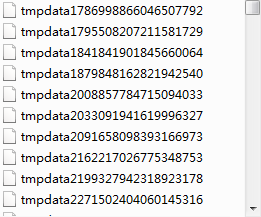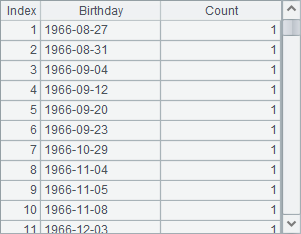• 集算器
教程
函数参考
习题
代码参考
用户参考
外部库使用指南
数据文件工具用户参考
DQL教程
集群管理器使用说明
SPL命令执行器用户参考
• 润乾报表
教程
填报教程
分析教程
报表中心教程
用户参考
程序员参考
高级指南
通用查询
DQL部署集成手册
• 易明建模
用户参考
json参数说明
• 润乾官网

# 组游标

## 按表达式分组取数

 A B 1 =file("Order_Wines.txt") 2 =file("Order_Electronics.txt") 3 =file("Order_Foods.txt") 4 =file("Order_Books.txt") 5 =[A1:A4].(~.cursor@t().sortx(PID)) 6 =A5.mergex(PID) 7 for 19 =A6.skip(;PID) 8 =A6.fetch(;PID) 9 >A6.close()

A6的游标中，包含着4种类型商品的销售数据，并按照PID排序。从A8中可以读出第20件商品的记录如下：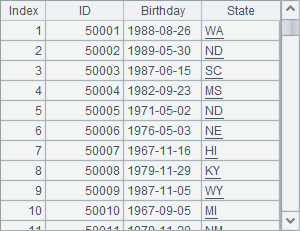## 文件游标中的分段读取

 A 1 =file("Order_Wines.txt") 2 =file("Order_Electronics.txt") 3 =file("Order_Foods.txt") 4 =file("Order_Books.txt") 5 =[A1:A4].(~.cursor@t().sortx(PID)) 6 =A5.mergex(PID) 7 =file("Order_Products.btx") 8 >A7.export@b(A6)

 A 1 =file("Order_Products.btx") 2 =A1.cursor@b(;1:100) 3 =A2.fetch() 4 =A1.cursor@b(;2:100) 5 =A4.fetch()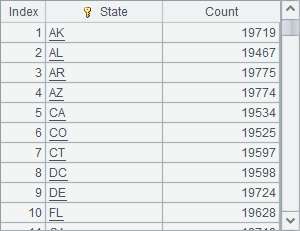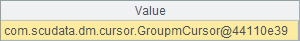A 1 =file("Order_Wines.txt") 2 =file("Order_Electronics.txt") 3 =file("Order_Foods.txt") 4 =file("Order_Books.txt") 5 =[A1:A4].(~.cursor@t().sortx(PID)) 6 =A5.mergex(PID) 7 =file("Order_Products_G.btx") 8 >A7.export@b(A6;PID)

 A 1 =file("Order_Products_G.btx ") 2 =A1.cursor@b(;1:100) 3 =A2.fetch() 4 =A1.cursor@b(;2:100) 5 =A4.fetch()